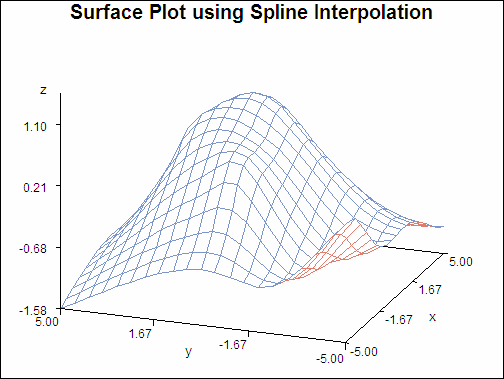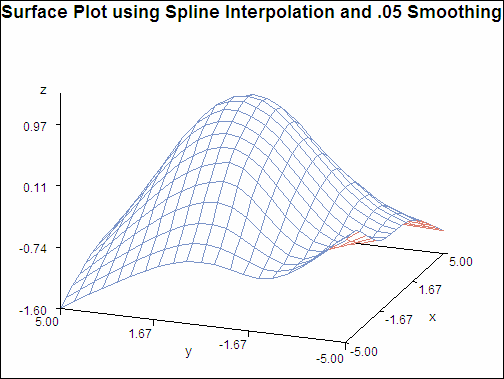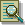The G3GRID Procedure

# Example 2: Spline and Smoothing Interpolations

Procedure features:

GRID statement options:

 SMOOTH= SPLINE
Data set: NUMS (see Using the Default Interpolation Method)
Sample library member: GTGSISS

This example extends Using the Default Interpolation Method to specify the SPLINE option on the GRID statement. The output data set, when used in PROC G3D, generates a smoother surface plot.

Surface Plot using Spline Interpolation (gtgsiss)The following plot extends Using the Default Interpolation Method to specify the SPLINE option, and the SMOOTH= option on the GRID statement. The SMOOTH= option is set to .05 for additional smoothing. The output data set, when used in PROC G3D, generates a smoother surface plot.

Surface Plot using Spline Interpolation and .05 Smoothing (gtgsiss)`goptions reset=all border;``title "Surface Plot using Spline Interpolation";````proc g3grid data=nums out=spline; grid y*x=z / spline axis1=-5 to 5 by .5 axis2=-5 to 5 by .5; run;``````proc g3d data=spline; plot y*x=z ; run; quit;````title "Surface Plot using Spline Interpolation and .05 Smoothing";````proc g3grid data=nums out=smoothed; grid y*x=z / spline smooth=.05 axis1=-5 to 5 by .5 axis2=-5 to 5 by .5; run; quit;``````proc g3d data=smoothed; plot y*x=z; run; quit;```Previous Page | Next Page | Top of Page